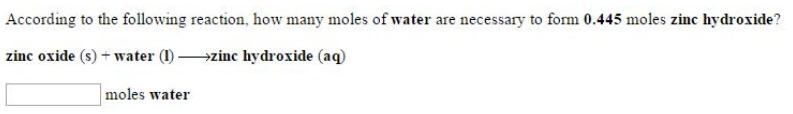# Problem: According to the following reaction, how many moles of water are necessary to form 0.445 moles zinc hydroxide? zinc oxide (s) + water (1) → zinc hydroxide (aq)

###### FREE Expert Solution
96% (63 ratings)###### Problem Details

According to the following reaction, how many moles of water are necessary to form 0.445 moles zinc hydroxide?

zinc oxide (s) + water (1) → zinc hydroxide (aq)